# Khan Engineering

We're the engineers behind Khan Academy. We're building a free, world-class education for anyone, anywhere.

## Latest posts

### Making Websites Work with Windows High Contrast Mode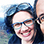Diedra Rater on March 21

### Kotlin for Python developersAasmund Eldhuset on Nov 29, 2018

### Using static analysis in Python, JavaScript and more to make your system safer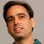Kevin Dangoor on Jul 26, 2018

### Kotlin on the server at Khan AcademyColin Fuller on Jun 28, 2018

### The Original Serverless Architecture is Still HereKevin Dangoor on May 31, 2018

### What do software architects at Khan Academy do?Kevin Dangoor on May 14, 2018

### New data pipeline management platform at Khan AcademyRagini Gupta on Apr 30, 2018

### Untangling our Python CodeCarter J. Bastian on Apr 16, 2018

### Slicker: A Tool for Moving Things in Python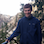Ben Kraft on Apr 2, 2018

### The Great Python Refactor of 2017 And Also 2018Craig Silverstein on Mar 19, 2018

### Working Remotely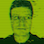Scott Grant on Oct 2, 2017

### Tips for giving your first code reviews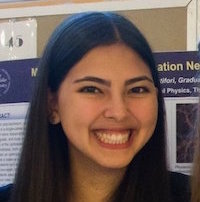Hannah Blumberg on Sep 18, 2017

### Let's Reduce! A Gentle Introduction to Javascript's Reduce Method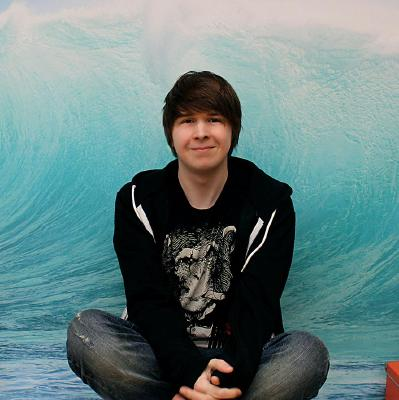Josh Comeau on Jul 10, 2017

### Creating Query Components with Apollo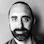Brian Genisio on Jun 12, 2017

### Migrating to a Mobile Monorepo for React Native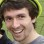Jared Forsyth on May 29, 2017

### Memcached-Backed Content InfrastructureBen Kraft on May 15, 2017

### Profiling App Engine MemcachedBen Kraft on May 1, 2017

### App Engine Flex Language ShootoutAmos Latteier on Apr 17, 2017

### What's New in OSS at Khan AcademyBrian Genisio on Apr 3, 2017

### Automating App Store ScreenshotsBryan Clark on Mar 27, 2017

### It's Okay to Break Things: Reflections on Khan Academy's Healthy Hackathon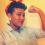Kimerie Green on Mar 6, 2017

### Interning at Khan Academy: from student to intern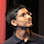Shadaj Laddad on Dec 12, 2016

### Prototyping with FramerNick Breen on Oct 3, 2016

### Evolving our content infrastructureWilliam Chargin on Sep 19, 2016

### Building a Really, Really Small Android App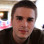Charlie Marsh on Aug 22, 2016

### A Case for Time Tracking: Data Driven Time-Management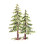Oliver Northwood on Aug 8, 2016

### Time Management at Khan AcademySeveral Authors on Jul 25, 2016

### Hackathons Can Be Healthy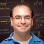Tom Yedwab on Jul 11, 2016

### Ensuring transaction-safety in Google App EngineCraig Silverstein on Jun 27, 2016

### The User Write Lock: an Alternative to Transactions for Google App EngineCraig Silverstein on Jun 20, 2016

### Khan Academy's Engineering PrinciplesBen Kamens on Jun 6, 2016

### Minimizing the length of regular expressions, in practiceCraig Silverstein on May 23, 2016

### Introducing SwiftTweaksBryan Clark on May 9, 2016

### The Autonomous Dumbledore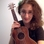Evy Kassirer on Apr 25, 2016

### Engineering career development at Khan Academy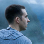Ben Eater on Apr 11, 2016

### Inline CSS at Khan Academy: Aphrodite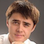Jamie Wong on Mar 29, 2016

### Starting Android at Khan Academy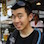Ben Komalo on Feb 29, 2016

### Automating Highly Similar Translations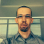Kevin Barabash on Feb 15, 2016

### The weekly snippet-server: open-sourcedCraig Silverstein on Feb 1, 2016

### Stories from our latest intern class2015 Interns on Dec 21, 2015

### Kanbanning the LearnStorm Dev ProcessKevin Dangoor on Dec 7, 2015

### Forgo JS packaging? Not so fastCraig Silverstein on Nov 23, 2015

### Switching to Slack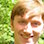Benjamin Pollack on Nov 9, 2015

### Receiving feedback as an intern at Khan AcademyDavid Wang on Oct 26, 2015

### Schrödinger's deploys no more: how we update translations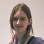Chelsea Voss on Oct 12, 2015

### i18nize-templates: Internationalization After the FactCraig Silverstein on Sep 28, 2015

### Making thumbnails fastWilliam Chargin on Sep 14, 2015

### Copy-pasting more than just text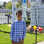Sam Lau on Aug 31, 2015

### No cheating allowed!!Phillip Lemons on Aug 17, 2015

### Fun with slope fields, css and reactMarcos Ojeda on Aug 5, 2015

### Khan Academy: a new employee's primer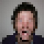Riley Shaw on Jul 20, 2015

### How wooden puzzles can destroy dev teams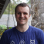John Sullivan on Jul 6, 2015

### Babel in Khan Academy's i18n ToolchainKevin Barabash on Jun 22, 2015

### tota11y - an accessibility visualization toolkit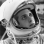Jordan Scales on Jun 8, 2015

# Making thumbnails fastby William Chargin on Sep 14, 2015

This article has a bit of exposition. The fun stuff starts in “Matrix magic.”

Video thumbnails are a great way to grab users’ attention. YouTube even provides guides for content creators about how to create compelling thumbnails. Until now, however, Khan Academy videos have always used the default YouTube thumbnails—just a screenshot of a video frame—and, given the nature of our content, these are almost always just black rectangles.

As part of our content library redesign initiative, we’re giving thumbnails a higher priority. We decided to enable our content creators to upload custom images and set custom title text for videos, and went through many design iterations before settling on a clean, bold, and eye-catching thumbnail design. Here are two examples: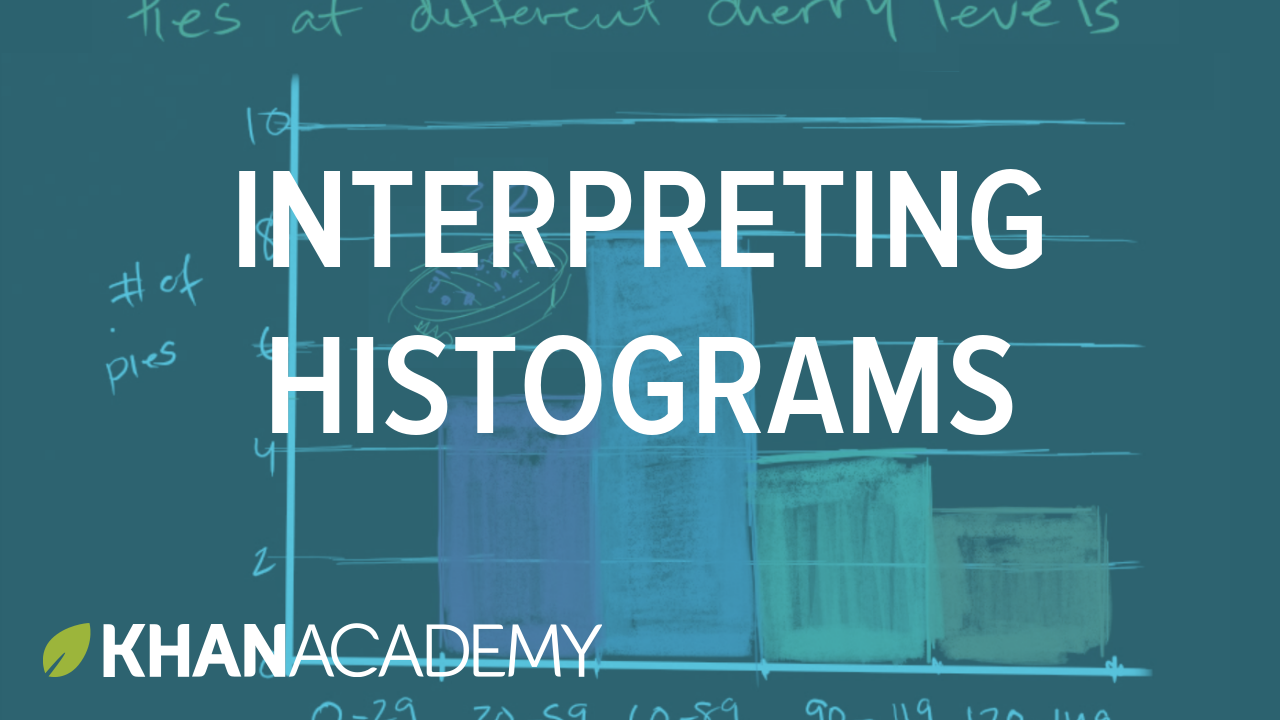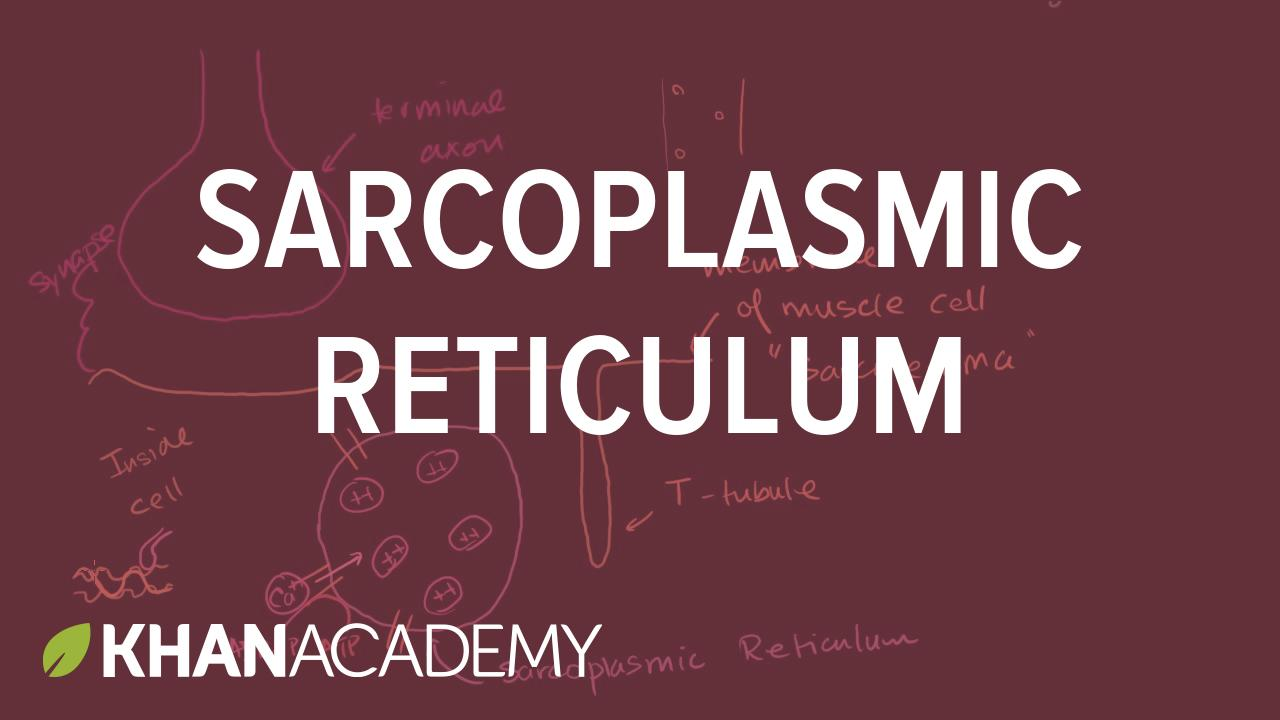The process for generating those thumbnail images from the source image (which you can see in the background) is as follows:

1. Resize the source image to fill $1280 \times 720$ pixels.

2. Desaturate the image by $50\%$.

3. Lighten the image by $50\%$.

4. Multiply the image with the third-darkest domain color (blue for math, red for science, etc.).

5. Overlay the text and Khan Academy logo.

You might be wondering what it means to “multiply” an image! The color data for each pixel of an image is stored in three components: red, green, and blue (RGB). To multiply two pixels, you multiply the values of their individual components, so $$(r_1, g_1, b_1) \cdot (r_2, g_2, b_2) = (r_1 \, r_2, g_1 \, g_2, b_1 \, b_2).$$

For example, yellow $(1, 1, 0)$ multiplied with light cyan $(\frac{1}{2}, 1, 1)$ yields greenish-yellow $(\frac{1}{2}, 1, 0)$.

The qualitative effects of the multiply filter are that black multiplied with anything stays black ($0x = 0$), white multiplied by anything doesn’t change the original color $(1x = x$), and other colors are tinted to match the multiply color.

Performing these kinds of image manipulations efficiently is essentially a solved problem. There are many freely available software toolkits that would be more than sufficient for our needs. However, our server infrastructure doesn't allow us to use those libraries, so we ended up implementing these operations ourselves. In the process, we learned a lot about how these operations really work, so we wanted to share that knowledge with you!

## How could we do this?

I had previously written some code to generate simpler thumbnails from a previous design, so I started by updating that code to generate the new thumbnails. The old code used PIL (the Python Imaging Library) for its image operations, because that’s the only such library supported by App Engine. In cases where PIL lacked functionality or produced unacceptably poor results, I had re-implemented some operations in numpy.

All three of the core operations we needed to perform—desaturate, lighten, and multiply—can be implemented on a per-pixel basis. That is, the output value of a pixel only depends on the original value for that pixel, not on any surrounding pixels (as with, e.g., a blur). PIL offers the PIL.Image.point(self, fn) function, which applies a given function to every pixel. At first glance, this seems perfect. However, further inspection reveals that this actually transforms each channel individually as well—that is, the value for the new red component can’t depend on the original green or blue. This would only work for lightening; it doesn’t provide enough information to work for desaturating or multiplying.

So, as before, I turned to numpy. I exported the image data to a 3D numpy array (such that arr[x][y][c] stores channel $c$ of pixel $(x, y)$). After searching the numpy docs, I found the apply_along_axis function, which let me apply a function to each pixel (the “$z$ axis” of my 3D array). For example, the following snippet averages the values of each component, so that $(r, g, b)$ becomes $(a, a, a)$, where $a = (r + g + b) / 3$:

import numpy as np
array = np.asarray(image);
transformed = np.apply_along_axis(
lambda px: np.ones(px.shape) * sum(px[:3]) / 3.0),
2,  # z-axis
array)


I created a simple function to transform any given pixel, and used apply_along_axis to apply it to the whole image. This approach worked.

It also took 35 seconds per thumbnail at $1280 \times 720$ pixels.

This was unacceptable.

## How could we do this better?

I profiled the code and determined that only a negligible part was being spent outside of core computations (under $20\%$). This meant that I wouldn’t be able to rely on Python-level optimizations to get the kind of speed we needed. So I started browsing the PIL documentation looking for ways to do this at a lower level. The ImageMath, ImageColor, and ImageFilter modules were no help. But then Andy discovered that PIL’s method for converting between color spaces allows the user to pass an arbitrary transformation matrix as an argument:

im.convert(mode, matrix) $\Rightarrow$ image

Converts an “RGB” image to “L” or “RGB” using a conversion matrix. The matrix is a 4- or 16-tuple.

Initially, I discounted this function because a 16-tuple would require a $4 \times 4$ transformation matrix, which didn’t seem appropriate for this use (I expected to use a $3 \times 4$ affine matrix). But then I looked more closely at the example:

rgb2xyz = (
0.412453, 0.357580, 0.180423, 0,
0.212671, 0.715160, 0.072169, 0,
0.019334, 0.119193, 0.950227, 0 )
out = im.convert("RGB", rgb2xyz)


The provided matrix was a 12-tuple, exactly as I wanted! So I looked at the PIL source and found, on line 767 of imaging.c,

if (!PyArg_ParseTuple(args, "s(ffffffffffff)", ...)) {
return NULL;
}


I’m no expert on Python’s C extensions, but there are clearly twelve fs there, and if that’s anything like the JNI interop, it means that the C code expects Python to pass it a 12-tuple of floats. This agrees with the example, and shows that the documentation is just wrong. (edit: The Pillow team has since fixed this! The documentation will be correct in new versions of Pillow.)

So this was good news! This meant that if I could express all our image manipulation in terms of affine transforms, we’d be able to use this function. I expected that this would be significantly faster, both because it’s running in C land and because it just has to perform a matrix multiplication on each pixel, and processors are really good at that.

## Matrix magic

This was the fun part. I needed to figure out what matrix to multiply the pixels by to create the proper transforms.

Heads up: This section assumes knowledge of matrix multiplication. If you want to learn the minimum necessary to proceed, check out our video on matrix–matrix multiplication. If you want to learn more about the underlying theory and why this works, check out our topic about matrix transformations, which discusses linear transformations! (Affine transformations are extension of linear transformations.)

For now, I’ll use the symbol $\otimes$ to represent multiplication of affine matrices. Later, we’ll explore how to actually compute this.

We need to do three different things to the image: desaturate, whiten, then multiply. Because matrix multiplication is associative, we can tackle these individually. I’ll cover the first one in depth and the other two more quickly.

### Desaturation

Suppose we have a single pixel with color $(r, g, b)$, which we’ll represent as a column vector $\begin{bmatrix} r & g & b \end{bmatrix}^T$. If you’re familiar with RGB colors, you’ll know that gray values are all of the form $\begin{bmatrix} k & k & k \end{bmatrix}^T$ for some $k$; that is, all the components are the same. A simple way to desaturate a color is to take the average of all the components and assign that to each component, as so: $$\begin{bmatrix} r \\ g \\ b \end{bmatrix} \mapsto \begin{bmatrix} (r + g + b) / 3 \\ (r + g + b) / 3 \\ (r + g + b) / 3 \end{bmatrix}.$$ This is a good start, but it doesn’t account for the fact that humans perceive green as much brighter than blue. Instead, the standard value for $k$ is given by a weighted average of the three components. That is, $$k = w_r r + w_g g + w_b b$$ for some $w_r, w_g, and w_b$ such that $w_r + w_g + w_b = 1$ (so as not to cause the image to get brighter or darker overall). The standard RGB weights are $w_r = 0.299$, $w_g = 0.587$, and $w_b = 0.114$. So we really want our desaturation transform to work as follows: $$\begin{bmatrix} r \\ g \\ b \end{bmatrix} \mapsto \begin{bmatrix} w_r r + w_g g + w_b b \\ w_r r + w_g g + w_b b \\ w_r r + w_g g + w_b b \end{bmatrix} = \begin{bmatrix} 0.299 r + 0.587 g + 0.114 b \\ 0.299 r + 0.587 g + 0.114 b \\ 0.299 r + 0.587 g + 0.114 b \end{bmatrix}.$$

Great. Now we know what we start with and what we want to end up with. At this point, we want to find a matrix $D$ such that $$D \otimes \begin{bmatrix} r \\ g \\ b \end{bmatrix} = \left[ \begin{array}{cccc} d_{11} & d_{12} & d_{13} & d_{14} \\ d_{21} & d_{22} & d_{23} & d_{24} \\ d_{31} & d_{32} & d_{33} & d_{34} \end{array} \right] \otimes\begin{bmatrix} r \\ g \\ b \end{bmatrix} = \begin{bmatrix} w_r r + w_g g + w_b b \\ w_r r + w_g g + w_b b \\ w_r r + w_g g + w_b b \end{bmatrix}.$$

Let’s consider the first entry of the target matrix. By the definition of affine matrix transformation, we must have $$d_{11} r + d_{12} g + d_{13} b + d_{14} = w_r r + w_g g + w_b b.$$ Because we want this to hold for all values of $r$, $g$, and $b$, we can use the classic method of comparing coefficients to see immediately that we must have $d_{11} = w_r$, $d_{12} = w_g$, $d_{13} = w_b$, and $d_{14} = 0$ (because there is no constant term on the right-hand side).

Similar logic applies to the other two rows, so we see that $$D = \left[ \begin{array}{cccc} w_r & w_g & w_b & 0 \\ w_r & w_g & w_b & 0 \\ w_r & w_g & w_b & 0 \end{array} \right].$$ Sweet!

But wait—the spec said that we only want to partially desaturate the image. So let’s find a matrix to desaturate a matrix by some factor $k_D$. (In particular, the spec lets $k_D = 0.5$.)

We can do this by linearly interpolating each color component. So if $r_0$ is the initial red value and $r_1$ is the fully desaturated red value, then $r = (1 - k_D) r_0 + k_D r_1$ is the red value desaturated by the factor $k_D$. That is, we now want $$\begin{bmatrix} r \\ g \\ b \end{bmatrix} \mapsto \begin{bmatrix} (1 - k_D) r + k_D (w_r r + w_g g + w_b b) \\ (1 - k_D) g + k_D (w_r r + w_g g + w_b b) \\ (1 - k_D) b + k_D (w_r r + w_g g + w_b b) \end{bmatrix}.$$ Similarly to before, we can create a new matrix $D$, and solve $$D \otimes \begin{bmatrix} r \\ g \\ b \end{bmatrix} = \left[ \begin{array}{ccc|c} d_{11} & d_{12} & d_{13} & d_{14} \\ d_{21} & d_{22} & d_{23} & d_{24} \\ d_{31} & d_{32} & d_{33} & d_{34} \end{array} \right] \otimes \begin{bmatrix} r \\ g \\ b \end{bmatrix}$$ $$= \begin{bmatrix} (1 - k_D) r + k_D (w_r r + w_g g + w_b b) \\ (1 - k_D) g + k_D (w_r r + w_g g + w_b b) \\ (1 - k_D) b + k_D (w_r r + w_g g + w_b b) \end{bmatrix}.$$ Let’s look at the first row. The right-hand side expands to $$(k_D w_r + 1 - k_D) r + k_D w_g g + k_D w_b b,$$ so we see that we must have $$d_{11} = k_D w_r + 1 - k_D, \quad d_{12} = k_D w_g, \quad \text{and} \quad d_{13} = k_D w_b.$$ In the second row, we similarly have $$d_{21} = k_D w_r, \quad d_{22} = k_D w_g + 1 - k_D, \quad \text{and} \quad d_{23} = k_D w_b.$$ Finally, in the third row, we similarly have $$d_{31} = k_D w_r, \quad d_{32} = k_D w_g, \quad \text{and} \quad d_{33} = k_D w_b + 1 - k_D.$$ As before, all the constant terms are zero. Therefore, the new desaturation matrix is $$D = \left[ \begin{array}{ccc|c} k_D w_r + (1 - k_D) & k_D w_g & k_D w_b & 0 \\ k_D w_r & k_D w_g + (1 - k_D) & k_D w_b & 0 \\ k_D w_r & k_D w_g & k_D w_b + (1 - k_D) & 0 \end{array} \right].$$ We can write this perhaps a bit more cleanly as $$D = \left[ \begin{array}{ccc|c} k_D w_r & k_D w_g & k_D w_b & 0 \\ k_D w_r & k_D w_g & k_D w_b & 0 \\ k_D w_r & k_D w_g & k_D w_b & 0 \end{array} \right] + (1 - k_D) I_{34},$$ if we interpret “$I_{34}$” as the identity affine matrix. Some might write this in terms of embedded matrices as $$D = \left[ \begin{array}{c|c} k_D \vec w & \\ k_D \vec w & \vec 0 \\ k_D \vec w & \end{array} \right] + (1 - k_D) \left[ \begin{array}{c|c} I_3 & \vec 0 \end{array} \right].$$

### Lightening

We next wanted to lighten the images by some factor $k_L = 0.5$. White has a value of $1$, so we can transform some channel $x$ to $k_L + (1 - k_L) x$. Therefore, we want to map $$\begin{bmatrix} r \\ g \\ b \end{bmatrix} \mapsto \begin{bmatrix} k_L + (1 - k_L) r \\ k_L + (1 - k_L) g \\ k_L + (1 - k_L) b \end{bmatrix}.$$ We can immediately see that the matrix will be diagonal, because none of the components depends on the others. We also see that each component is scaled by a factor $1 - k_L$, and then a constant factor $k_L$ is added. (If you don’t see this, you can go through the same process as with desaturation to convince yourself.) So the lightening matrix must be $$L = \left[ \begin{array}{ccc|c} 1 - k_L & & & k_L \\ & 1 - k_L & & k_L \\ & & 1 - k_L & k_L \end{array} \right] = \left[ \begin{array}{c|c} (1 - k_L) I_3 & k_L \end{array} \right].$$ That wasn’t too bad at all!

### Multiplying

Finally, we want to multiply our color with some base color $\vec c = \begin{bmatrix} c_r & c_g & c_b \end{bmatrix}^T$. The definition of RGB image multiplication stipulates that we multiply each of three channels individually, so that $$\begin{bmatrix} r \\ g \\ b \end{bmatrix} \mapsto \begin{bmatrix} c_r r \\ c_g g \\ c_b b \end{bmatrix}.$$ In this case, a full-factor multiply was specified, so we don’t need to interpolate anything. Therefore, the matrix is diagonal: $$M = \left[ \begin{array}{ccc|c} c_r & & & 0 \\ & c_g & & 0 \\ & & c_b & 0 \end{array} \right] = \left[ \begin{array}{c|c} \mathrm{diag} \ \vec c & 0 \end{array} \right].$$

## Combining the matrices

Now that I had the three transformation matrices that I needed, I could have applied transforms three times. But hold on a moment: applying these transforms is just the same as multiplying matrices, which is associative.

Suppose that our initial pixel is a column vector $\vec p_0$. To desaturate it, we left-multiply by a slightly-modified version of the desaturation matrix, which I’ll denote $D^*$; that is, $\vec p_1 = D^* \, \vec p_0$ is the desaturated pixel. Then, we want to lighten this, so $\vec p_2 = L^* \vec p_1$. Finally, multiplying, $\vec p_3 = M^* \vec p_2$, and $\vec p_3$ is our final value.

But we can just substitute to show that $$\vec p_3 = M^* \vec p_2 = M^* (L^* \vec p_1) = M^* (L^* (D^* \vec p_0)) = (M^* L^* D^*) \vec p_0.$$ This shows that we can create a combined transform by just multiplying the matrices in reverse order! By pre-computing that matrix $M^* L^* D^*$, we only have to perform one matrix multiplication (per pixel) instead of three! If you ever wondered why the definition of matrix multiplication seems so convoluted, it’s because that definition allows awesome things like this to happen.

If you’re following along closely, you might note that the three matrices $M$, $L$, and $D$ are all $3 \times 4$ matrices. That poses a problem, because we can’t multiply them directly. This is where the starred variants come in. If $A$ is a $3 \times 4$ matrix representing an affine transformation, then we can let $$A^* = \begin{bmatrix} a_{11} & a_{12} & a_{13} & a_{14} \\ a_{21} & a_{22} & a_{23} & a_{24} \\ a_{31} & a_{32} & a_{33} & a_{34} \\ 0 & 0 & 0 & 1 \end{bmatrix}.$$ I claim that if we also extend the pixel vectors to have an extra $1$ entry at the bottom, then these matrices will behave as we desire under multiplication.

Let’s first take a look at just applying such a matrix to make sure it does what we want. Note that $$\begin{array}{rl} A^* \vec p &= \begin{bmatrix} a_{11} & a_{12} & a_{13} & a_{14} \\ a_{21} & a_{22} & a_{23} & a_{24} \\ a_{31} & a_{32} & a_{33} & a_{34} \\ 0 & 0 & 0 & 1 \end{bmatrix} \begin{bmatrix} r \\ g \\ b \\ 1 \end{bmatrix} \\ \\ &= \begin{bmatrix} a_{11} r + a_{12} g + a_{13} b + a_{14} (1) \\ a_{21} r + a_{22} g + a_{23} b + a_{24} (1) \\ a_{31} r + a_{32} g + a_{33} b + a_{34} (1) \\ 0r + 0g + 0b + 1(1) \end{bmatrix} \\ \\ &= \begin{bmatrix} a_{11} r + a_{12} g + a_{13} b + a_{14} \\ a_{21} r + a_{22} g + a_{23} b + a_{24} \\ a_{31} r + a_{32} g + a_{33} b + a_{34} \\ 1 \end{bmatrix}, \end{array}$$ which is exactly what we expect: the “normal matrix” part multiplied as normal, the affine components were added as constants, and we even get a $1$ back in the last entry so that we can do this again the next time we multiply!

It’s also not hard to show that the matrices compose as desired, but I’ll omit that here because it involves adding a lot of things and your eyes would just glaze over anyway. It’ll be more meaningful if you do it yourself.

In this way, we can create the three original matrices, then their starred variants, then multiply them together to get a single matrix that we can apply to each pixel.

## Code?

Sure!

The implementation is a tad different because color values are integers in $\mathbb Z \cap [0, 256)$ instead of arbitrary reals in the interval $[0, 1]$. But the core idea is the same.

## Results

The time to composite a full-resolution ($1280 \times 720$) thumbnail using this method is $25.8\,\mathrm{ms}$ (on my laptop). The most time-consuming factors for the request are now network overhead, middleware, and RPC calls (e.g., to load the actual image from Google Cloud Storage).

To put that in perspective, it’s a speedup of over $1350{\times}$.

This was acceptable.

## Lessons

• Don’t trust the documentation. (The compiler won’t.)

• Pay attention in math class (or on Khan Academy if your math class, like mine, didn’t cover this).

• For computationally intensive operations, low-level operations are a must. To go fast, do less.